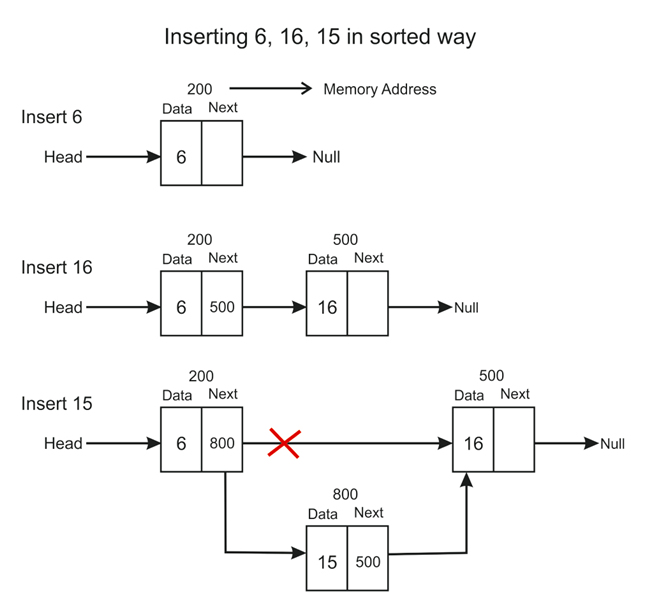# Insert nodes in a linked list in a sorted way (Ascending Order)

## In this algorithm, we will insert elements in the linkedlist in a sorted way ie, data values from low to high in ascending order

### Example## Algorithm

1. If the head node is Null, then insert the data in the head node.
2. else, if the input data is less than the start node, then insert the node at the start
3. if the input data is greater than the start node, till you get the right position to insert, move the     temporary pointer. If the temporary pointer's next value is null, then insert the node at the end.
4. If the element lies between any two values, then connect the node to the previous node and the next node ie, t->next = temp->next and temp->next = t.

## C++ Program

``````#include <bits/stdc++.h>
using namespace std;

struct LL{
int data;
LL *next;
};

void sortedInsert(struct LL**head , int X)
{
LL *t = new LL;
//if list is empty
{
}

else
{

if(X < temp->data) //start node
{
t = new LL;
t->data = X;
}

else
{
while(temp->next != NULL and !(X < temp->next->data && X >= temp->data)) //as it is sorted so X must lie between the consecutive values or else at end
temp=temp->next;

if(temp->next == NULL) //X will go to end
{
temp->next = new LL;
temp = temp->next;
temp->data = X;
temp->next = NULL;
}
else //X is inserted in between some nodes in list
{
t = new LL;
t->data = X;
t->next = temp->next; //make the new node's next as the next of current node because the 't' node will lie between consecutive nodes
temp->next = t;
}
}
}
}

{
while(temp!=NULL)
{
if(temp->next!=NULL)
cout<<temp->data<<" ->";
else
cout<<temp->data;

temp=temp->next; //move to next node
}
//O(number of nodes)
cout<<endl;
}

int main()
{

struct LL *head = NULL; //initial list has no elements
cout<<"\nCurrent List is :-\n";
cout<<"\nCurrent List is :-\n";
cout<<"\nCurrent List is :-\n";
cout<<"\nCurrent List is :-\n";
cout<<"\nCurrent List is :-\n";